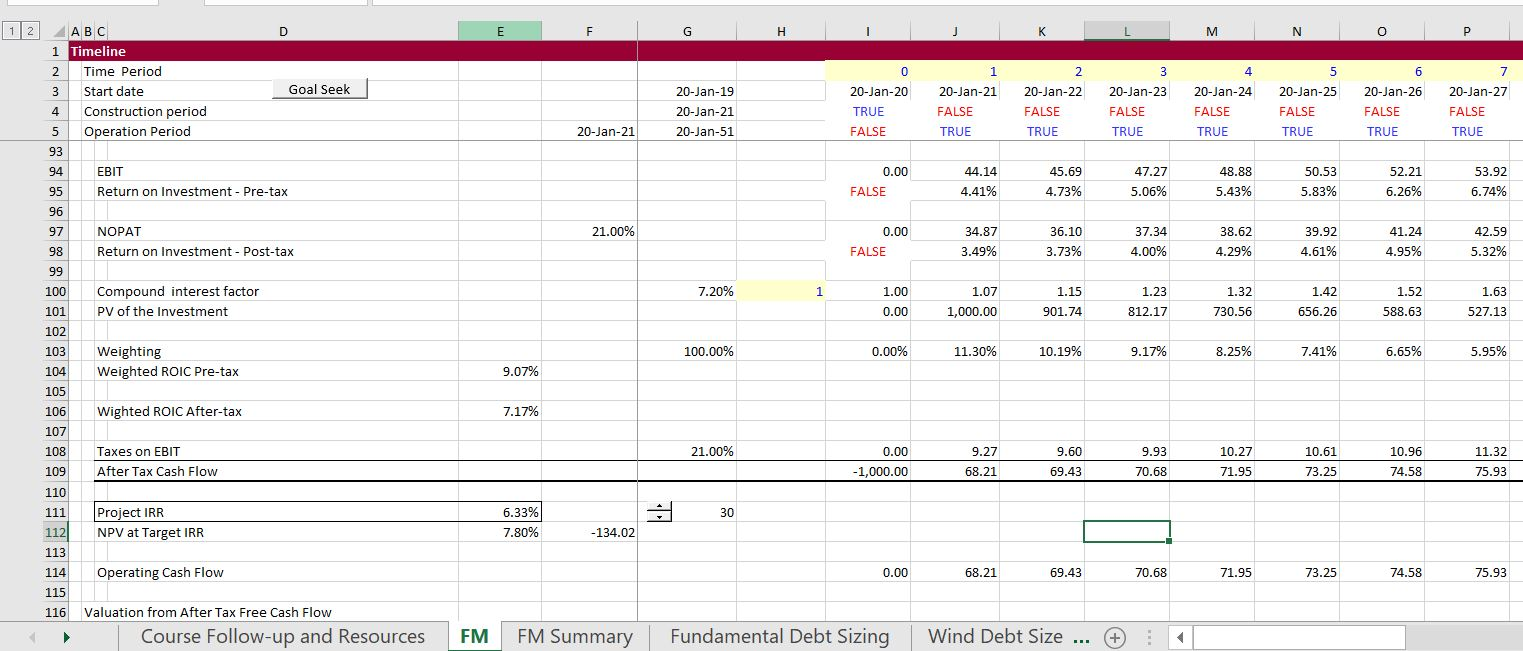# Alternative to IRR – PV of Cash Flow Relative to PV of Investment

This webpage discusses an alternative to the IRR that measures the PV of the future cash flows divided by the investment (or the PV of the capital expenditures plus other costs).  This measure is analogous to the price to book ratio or the EV/Invested capital ratio used in corporate finance.  The ratio can be used to gauge the performance of an existing asset after the COD.  As the measure is not in terms of a simple rate of return or growth rate, this website describes how it can be interpreted.  As other measures that are alternative to the IRR, the statistic does require an estimate of the cost of capital.

## Mechanics of Value to Investment Ratio

When computing cash flow for the project or for the equity investors, you can separate the negative value of the investment from the positive cash flow. Given a discount rate, you can compute the present value of both the positive inflows and you can also compute the present value of the investment. The ratio is the value of positive inflows divided by the present value of the outflows.  When you compute the ratio for an existing investment, you should use the economic depreciation and derive the economic value of the investment from the economic depreciation.

## Interpretation of Value to Investment Ratio

To see how the value to investment ratio works, you can use some financial mathematics formulas.  The formula for the market value to the investment ratio in general is: Value/BV = (ROE-g)/(k-g).  This can be demonstrated as follows:

• Value = D1/(k-g)
• g = ROE * (1-DPO)
• (1-DPO) = g/ROE
• DPO = 1-g/ROE
• E1 = ROE * BV

• Value = ROE * BV * (1-g/ROE)/(k-g)
• Value = (BV * ROE – g * BV * ROE/ROE)/(k-g)
• Value = BV * (ROE – g)/(k-g)
• Value/BV = (ROE – g)/(k-g)

In project finance, we could assume that g = 0 and the that the formula reduces to:

Value/BV = IRR/Cost of Capital

This means for example, that if the IRR is twice the cost of capital, the ratio will be 2.0.  If the return is 15% and the cost of capital is 10%, then the ratio is 1.5.  When the return is the same as the cost of capital, the ratio is 1.0.